# RD Sharma Solutions for Class 6 Maths Chapter 20: Mensuration Objective Type Questions

This exercise contains objective type questions at the end covering topics from the entire Chapter. The solutions contain brief explanations in order to make them easier for the students to understand from the exam point of view. Here, important formulas and topics are highlighted to help students grasp the concepts. The students can gain knowledge on how much they have understood across all concepts. To know more about the important concepts which are covered here, RD Sharma Solutions Class 6 Maths Chapter 20 Mensuration Objective Type Questions are available for free download.

## RD Sharma Solutions for Class 6 Maths Chapter 20: Mensuration Objective Type Questions Download PDF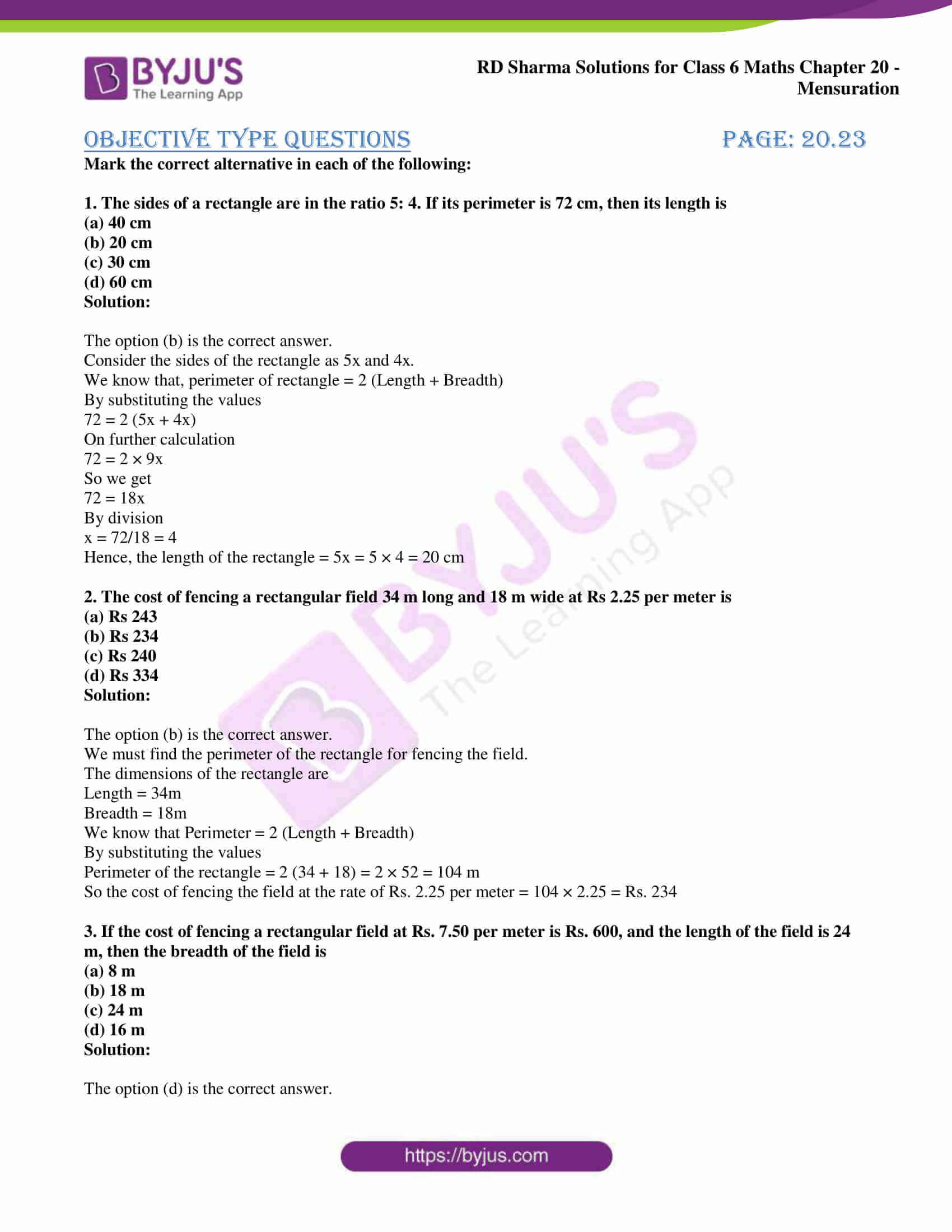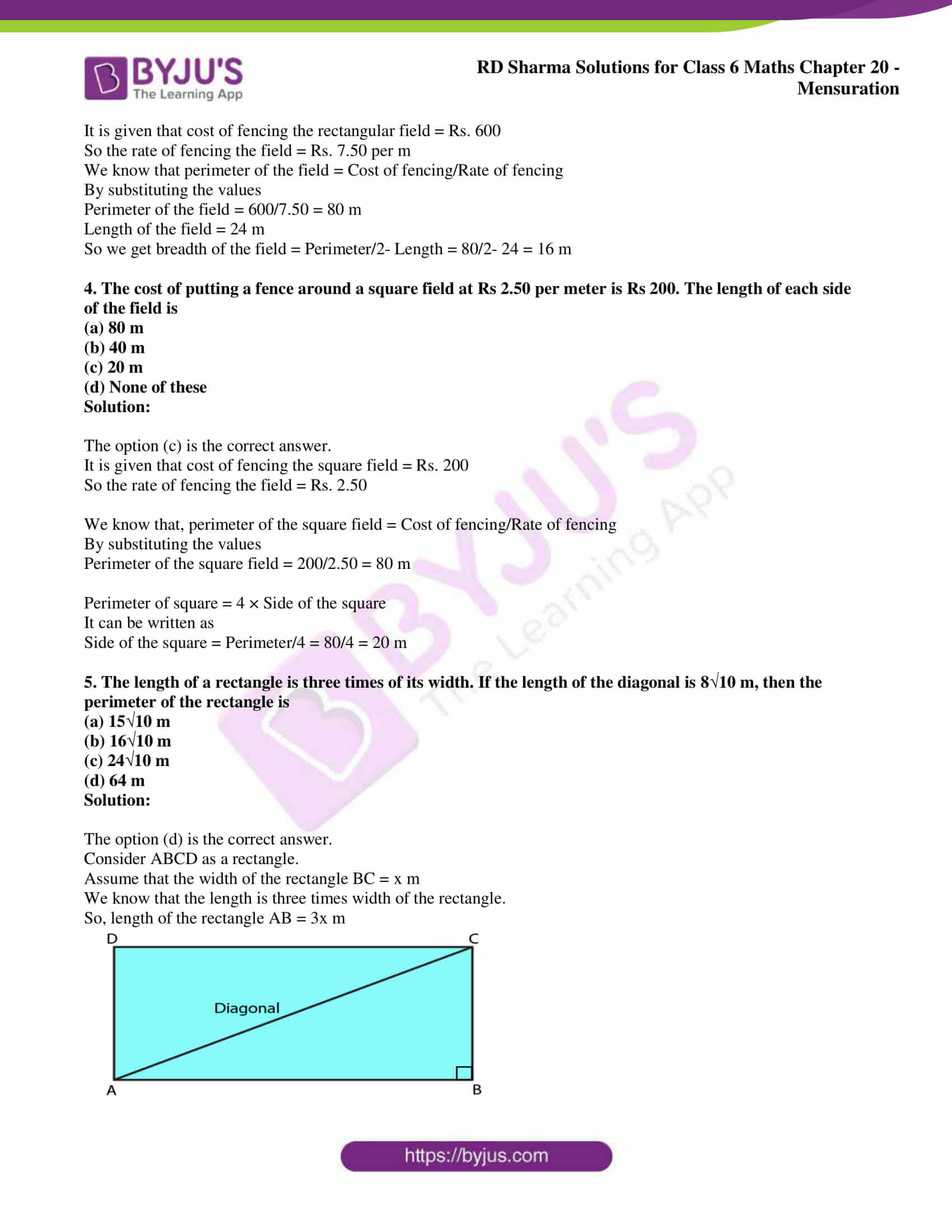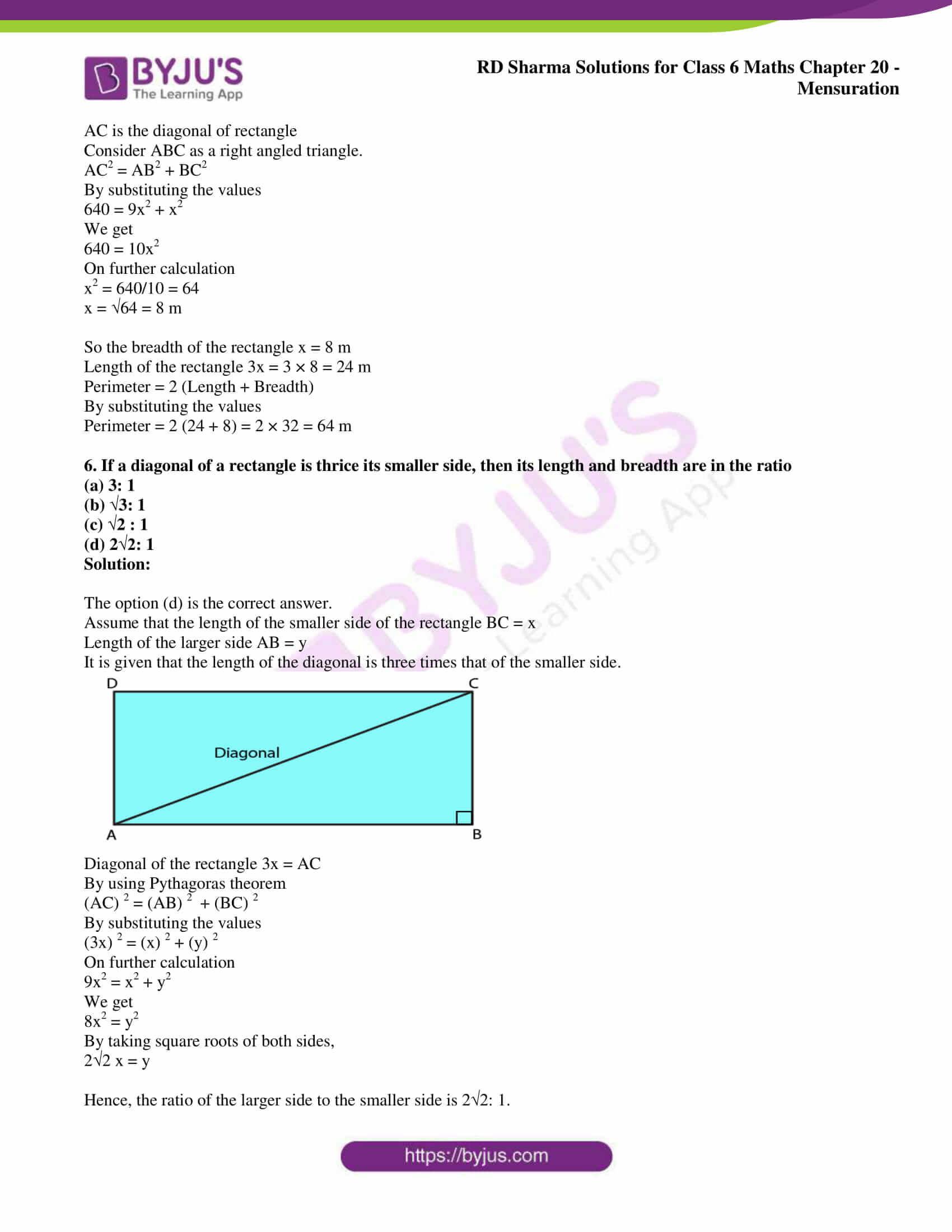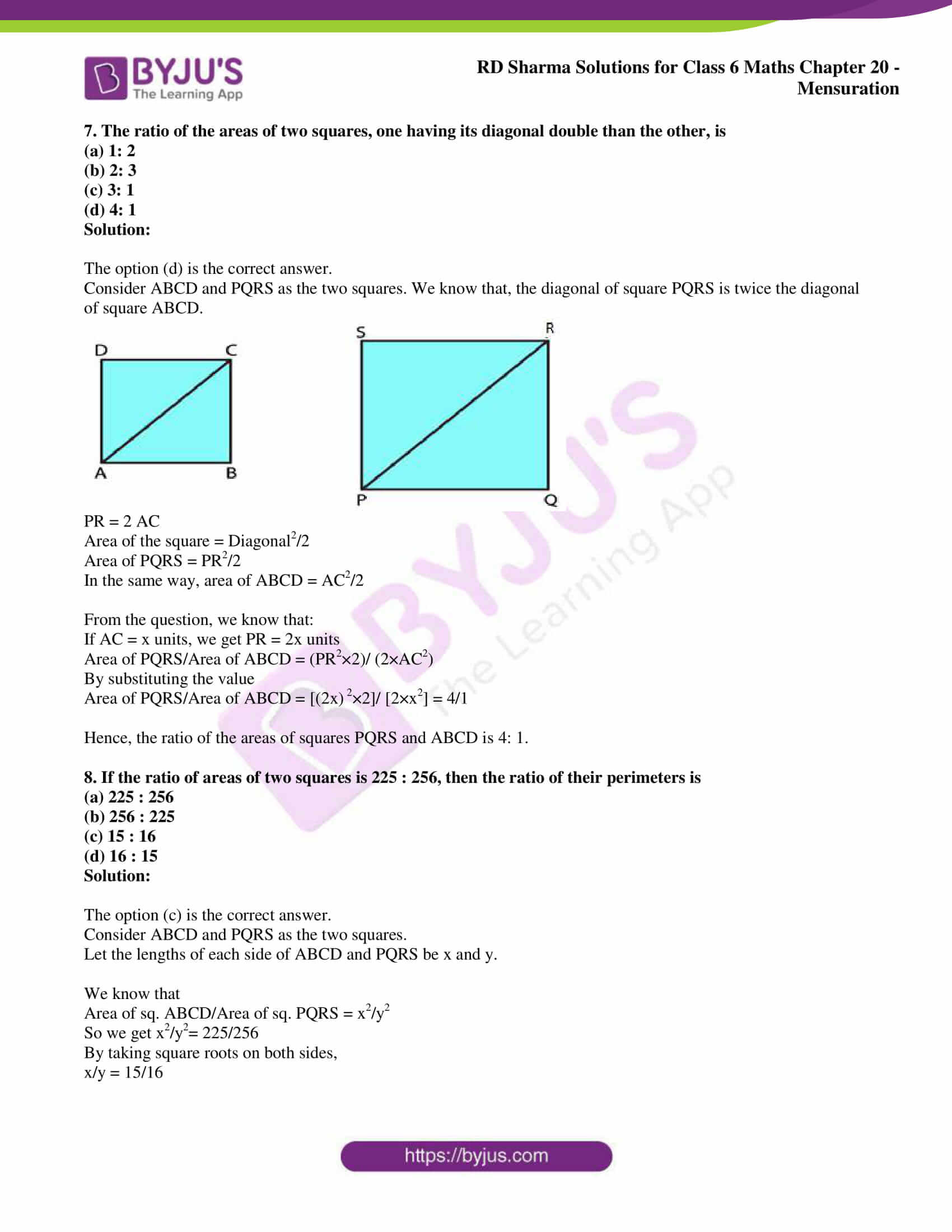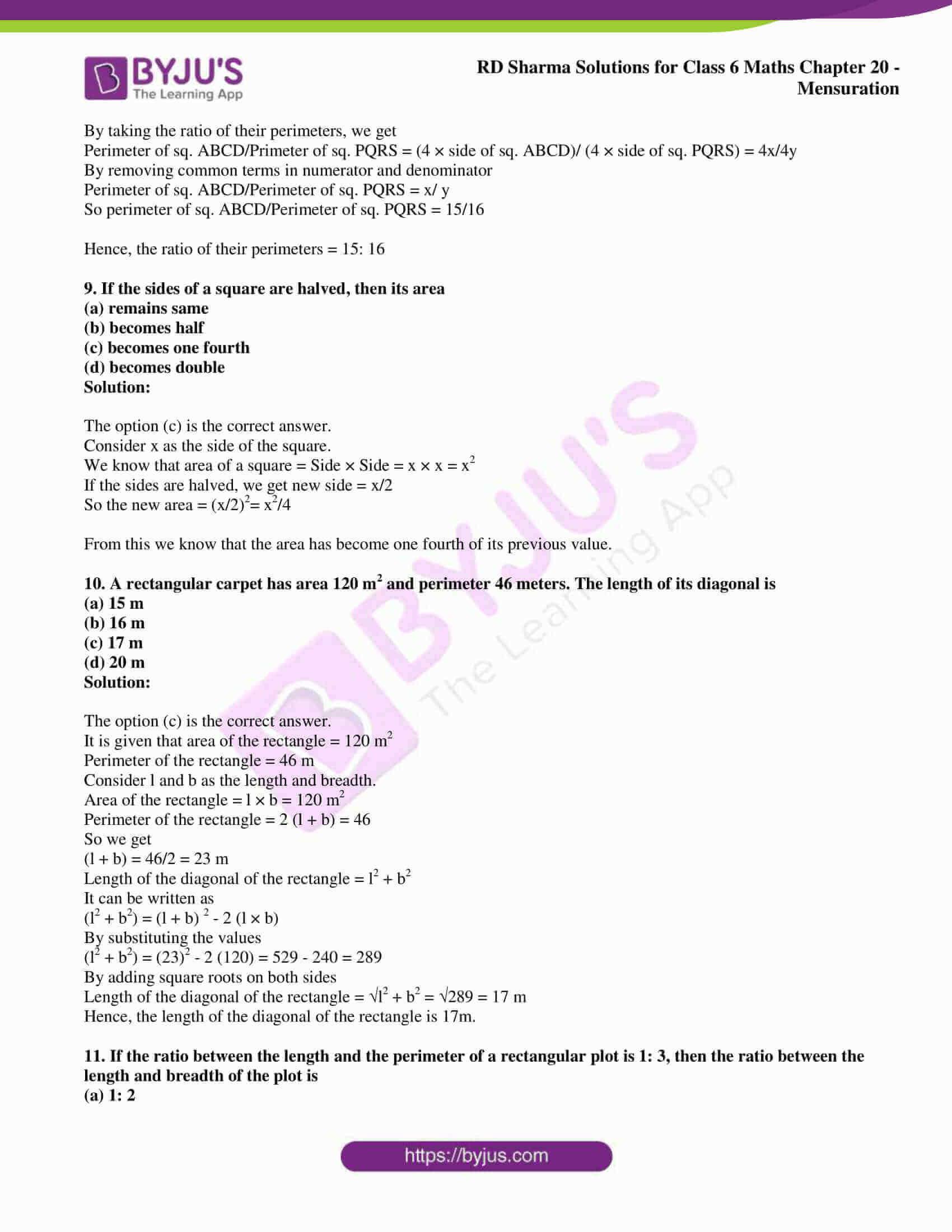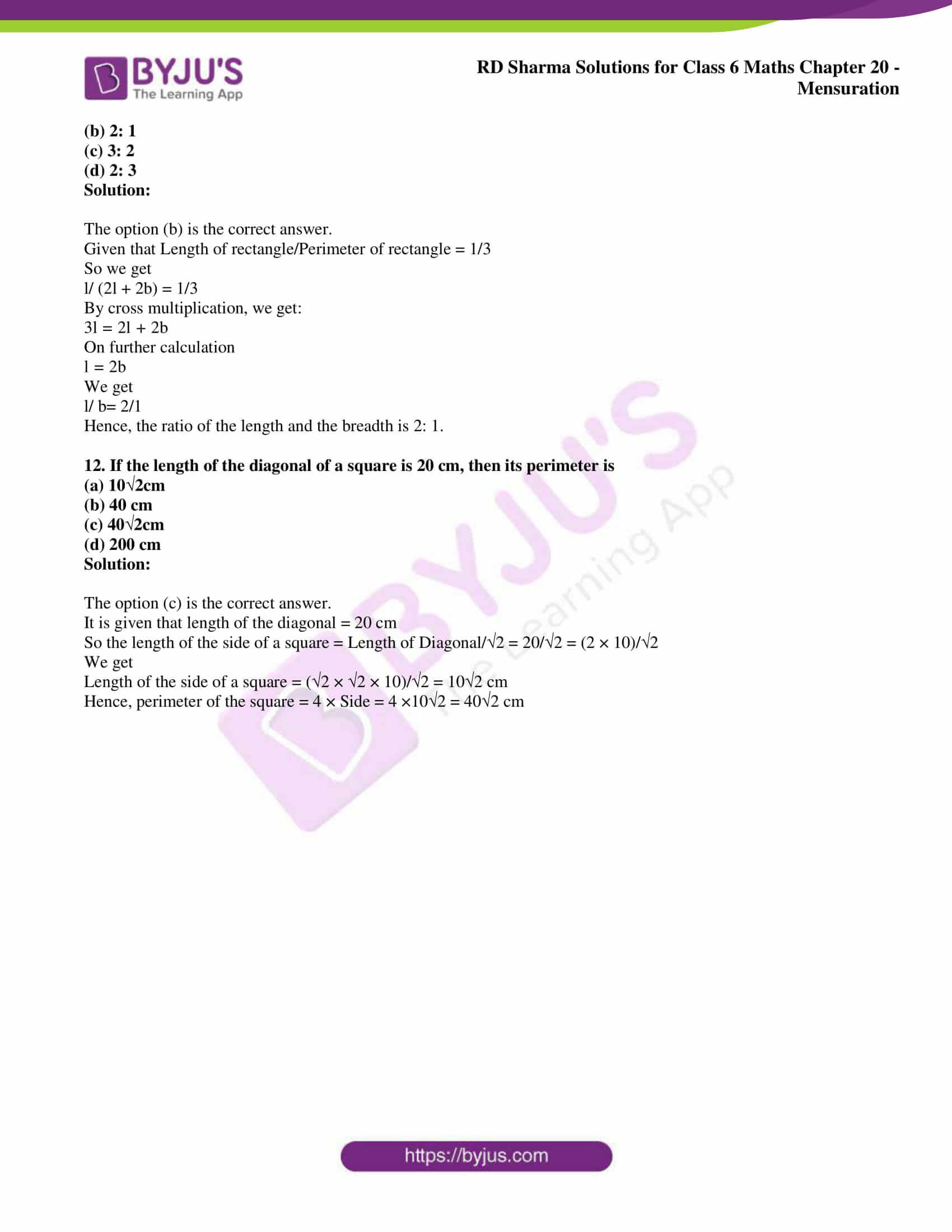### Objective Type Questions page: 20.23

Mark the correct alternative in each of the following:

1. The sides of a rectangle are in the ratio 5: 4. If its perimeter is 72 cm, then its length is
(a) 40 cm
(b) 20 cm
(c) 30 cm
(d) 60 cm

Solution:

The option (b) is the correct answer.

Consider the sides of the rectangle as 5x and 4x.

We know that, perimeter of rectangle = 2 (Length + Breadth)

By substituting the values

72 = 2 (5x + 4x)

On further calculation

72 = 2 × 9x

So we get

72 = 18x

By division

x = 72/18 = 4

Hence, the length of the rectangle = 5x = 5 × 4 = 20 cm

2. The cost of fencing a rectangular field 34 m long and 18 m wide at Rs 2.25 per meter is
(a) Rs 243
(b) Rs 234
(c) Rs 240
(d) Rs 334

Solution:

The option (b) is the correct answer.

We must find the perimeter of the rectangle for fencing the field.

The dimensions of the rectangle are

Length = 34m

We know that Perimeter = 2 (Length + Breadth)

By substituting the values

Perimeter of the rectangle = 2 (34 + 18) = 2 × 52 = 104 m

So the cost of fencing the field at the rate of Rs. 2.25 per meter = 104 × 2.25 = Rs. 234

3. If the cost of fencing a rectangular field at Rs. 7.50 per meter is Rs. 600, and the length of the field is 24 m, then the breadth of the field is
(a) 8 m
(b) 18 m
(c) 24 m
(d) 16 m

Solution:

The option (d) is the correct answer.

It is given that cost of fencing the rectangular field = Rs. 600

So the rate of fencing the field = Rs. 7.50 per m

We know that perimeter of the field = Cost of fencing/Rate of fencing

By substituting the values

Perimeter of the field = 600/7.50 = 80 m

Length of the field = 24 m

So we get breadth of the field = Perimeter/2- Length = 80/2- 24 = 16 m

4. The cost of putting a fence around a square field at Rs 2.50 per meter is Rs 200. The length of each side of the field is
(a) 80 m
(b) 40 m
(c) 20 m
(d) None of these

Solution:

The option (c) is the correct answer.

It is given that cost of fencing the square field = Rs. 200

So the rate of fencing the field = Rs. 2.50

We know that, perimeter of the square field = Cost of fencing/Rate of fencing

By substituting the values

Perimeter of the square field = 200/2.50 = 80 m

Perimeter of square = 4 × Side of the square

It can be written as

Side of the square = Perimeter/4 = 80/4 = 20 m

5. The length of a rectangle is three times of its width. If the length of the diagonal is 8√10 m, then the perimeter of the rectangle is
(a) 15√10 m
(b) 16√10 m
(c) 24√10 m
(d) 64 m

Solution:

The option (d) is the correct answer.

Consider ABCD as a rectangle.

Assume that the width of the rectangle BC = x m

We know that the length is three times width of the rectangle.

So, length of the rectangle AB = 3x m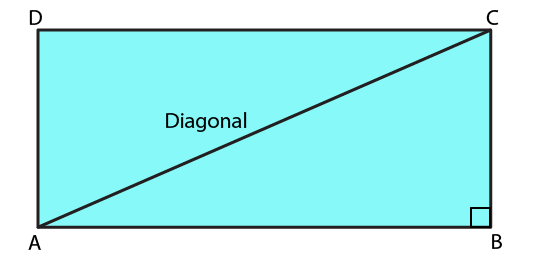AC is the diagonal of rectangle

Consider ABC as a right angled triangle.

AC2 = AB2 + BC2

By substituting the values

640 = 9x2 + x2

We get

640 = 10x2

On further calculation

x2 = 640/10 = 64

x = √64 = 8 m

So the breadth of the rectangle x = 8 m

Length of the rectangle 3x = 3 × 8 = 24 m

Perimeter = 2 (Length + Breadth)

By substituting the values

Perimeter = 2 (24 + 8) = 2 × 32 = 64 m

6. If a diagonal of a rectangle is thrice its smaller side, then its length and breadth are in the ratio
(a) 3: 1
(b) √3: 1
(c) √2 : 1
(d) 2√2: 1

Solution:

The option (d) is the correct answer.

Assume that the length of the smaller side of the rectangle BC = x

Length of the larger side AB = y

It is given that the length of the diagonal is three times that of the smaller side.Diagonal of the rectangle 3x = AC

By using Pythagoras theorem

(AC) 2 = (AB) 2   + (BC) 2

By substituting the values

(3x) 2 = (x) 2 + (y) 2

On further calculation

9x2 = x2 + y2

We get

8x2 = y2

By taking square roots of both sides,

2√2 x = y

Hence, the ratio of the larger side to the smaller side is 2√2: 1.

7. The ratio of the areas of two squares, one having its diagonal double than the other, is
(a) 1: 2
(b) 2: 3
(c) 3: 1
(d) 4: 1

Solution:

The option (d) is the correct answer.

Consider ABCD and PQRS as the two squares. We know that, the diagonal of square PQRS is twice the diagonal of square ABCD.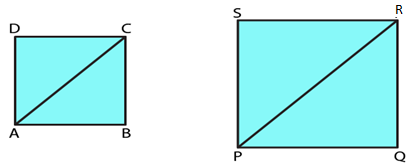PR = 2 AC

Area of the square = Diagonal2/2

Area of PQRS = PR2/2

In the same way, area of ABCD = AC2/2

From the question, we know that:

If AC = x units, we get PR = 2x units

Area of PQRS/Area of ABCD = (PR2×2)/ (2×AC2

By substituting the value

Area of PQRS/Area of ABCD = [(2x) 2×2]/ [2×x2] = 4/1

Hence, the ratio of the areas of squares PQRS and ABCD is 4: 1.

8. If the ratio of areas of two squares is 225 : 256, then the ratio of their perimeters is
(a) 225 : 256
(b) 256 : 225
(c) 15 : 16
(d) 16 : 15

Solution:

The option (c) is the correct answer.

Consider ABCD and PQRS as the two squares.

Let the lengths of each side of ABCD and PQRS be x and y.

We know that

Area of sq. ABCD/Area of sq. PQRS = x2/y2

So we get x2/y2= 225/256

By taking square roots on both sides,

x/y = 15/16

By taking the ratio of their perimeters, we get

Perimeter of sq. ABCD/Primeter of sq. PQRS = (4 × side of sq. ABCD)/ (4 × side of sq. PQRS) = 4x/4y

By removing common terms in numerator and denominator

Perimeter of sq. ABCD/Perimeter of sq. PQRS = x/ y

So perimeter of sq. ABCD/Perimeter of sq. PQRS = 15/16

Hence, the ratio of their perimeters = 15: 16

9. If the sides of a square are halved, then its area
(a) remains same
(b) becomes half
(c) becomes one fourth
(d) becomes double

Solution:

The option (c) is the correct answer.

Consider x as the side of the square.

We know that area of a square = Side × Side = x × x = x2

If the sides are halved, we get new side = x/2

So the new area = (x/2)2= x2/4

From this we know that the area has become one fourth of its previous value.

10. A rectangular carpet has area 120 m2 and perimeter 46 meters. The length of its diagonal is
(a) 15 m
(b) 16 m
(c) 17 m
(d) 20 m

Solution:

The option (c) is the correct answer.

It is given that area of the rectangle = 120 m2

Perimeter of the rectangle = 46 m

Consider l and b as the length and breadth.

Area of the rectangle = l × b = 120 m2

Perimeter of the rectangle = 2 (l + b) = 46

So we get

(l + b) = 46/2 = 23 m

Length of the diagonal of the rectangle = l2 + b2

It can be written as

(l2 + b2) = (l + b) 2 – 2 (l × b)

By substituting the values

(l2 + b2) = (23)2 – 2 (120) = 529 – 240 = 289

By adding square roots on both sides

Length of the diagonal of the rectangle = √l2 + b2 = √289 = 17 m

Hence, the length of the diagonal of the rectangle is 17m.

11. If the ratio between the length and the perimeter of a rectangular plot is 1: 3, then the ratio between the length and breadth of the plot is
(a) 1: 2
(b) 2: 1
(c) 3: 2
(d) 2: 3

Solution:

The option (b) is the correct answer.

Given that Length of rectangle/Perimeter of rectangle = 1/3

So we get

l/ (2l + 2b) = 1/3

By cross multiplication, we get:

3l = 2l + 2b

On further calculation

l = 2b

We get

l/ b= 2/1

Hence, the ratio of the length and the breadth is 2: 1.

12. If the length of the diagonal of a square is 20 cm, then its perimeter is
(a) 10√2cm
(b) 40 cm
(c) 40√2cm
(d) 200 cm

Solution:

The option (c) is the correct answer.

It is given that length of the diagonal = 20 cm

So the length of the side of a square = Length of Diagonal/√2 = 20/√2 = (2 × 10)/√2

We get

Length of the side of a square = (√2 × √2 × 10)/√2 = 10√2 cm

Hence, perimeter of the square = 4 × Side = 4 ×10√2 = 40√2 cm